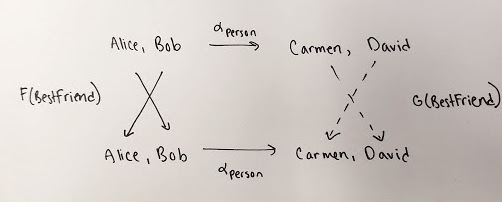Great, Sophie!

The most important puzzle in this lecture, toward our goal of understanding databases, is Puzzle 148. In [comment #14](https://forum.azimuthproject.org/discussion/comment/19536/#Comment_19536), Sophie gave an example of two naturally isomorphic databases built using the same schema:The rough idea is that when we have two naturally isomorphic databases, the _names_ of things may be different, but the _relationships_ between things are the same! We've got a category \$$\mathcal{C}\$$ with one object \$$\textrm{Person}\$$ and morphisms given by

$1_{\textrm{Person}} : \textrm{Person} \to \textrm{Person}$

$\textrm{BestFriend} : \textrm{Person} \to \textrm{Person}$

$\textrm{BestFriend} \circ \textrm{BestFriend} : \textrm{Person} \to \textrm{Person}$

$\textrm{BestFriend} \circ \textrm{BestFriend} \circ \textrm{BestFriend}: \textrm{Person} \to \textrm{Person}$

and so on, forever. This _abstractly_ describes the idea of people and their best friends. It's a **database schema**.

Then Sophie considers two databases built using this scheman. In other words, two functors

$F, G : \mathcal{C} \to \mathbf{Set} .$

Each of these assigns a specific _set_ to the object \$$\textrm{Person}\$$ and a specific function to the morphism \$$\textrm{BestFriend}\$$. The first has

$F(\textrm{Person}) = \lbrace \textrm{Alice}, \textrm{Bob} \rbrace$

and

$F(\textrm{BestFriend}) (\textrm{Alice}) = \textrm{Bob} , \qquad F(\textrm{BestFriend}) (\textrm{Bob}) = \textrm{Alice} .$

So, it describes a _concrete_ situation involving people and their best friends, where Alice is Bob's best friend and vice versa.

The second has

$G(\textrm{Person}) = \lbrace \textrm{Carmen}, \textrm{David} \rbrace$

and

$G(\textrm{BestFriend}) (\textrm{Carmen}) = \textrm{David} , \qquad G(\textrm{BestFriend}) (\textrm{David}) = \textrm{Carmen} .$

This is different than \$$F\$$, but it's 'the same in a way': it again describes a concrete situation where two people are each other's best friend!

We can make this 'the same in a way' idea precise using a natural isomorphism \$$\alpha : F \Rightarrow G \$$. This gives a bijection

$\alpha_{\textrm{Person}} : F(\textrm{Person}) \to G(\textrm{Person})$

obeying the naturality condition

$\alpha_{\textrm{Person}} \circ F(\textrm{BestFriend}) = G(\textrm{BestFriend}) \circ \alpha_{\textrm{Person}} .$

This naturality condition is the crucial thing to understand! It says

> If \$$x\$$ is \$$y\$$'s best friend in the first database, and \$$\alpha_{\textrm{Person}} x = x'\$$ and \$$\alpha_{\textrm{Person}}y = y'\$$, then \$$x'\$$ is \$$y'\$$'s best friend in the second database.

For example:

> If Alice is Bob's best friend in the first database, and Alice is mapped to Carmen and Bob is mapped to David, then Carmen must be David's best friend in the second database.

Get it?

Here are some followup puzzles... for people other than Sophie:

**Puzzle.** With the same choice of \$$F\$$ and \$$G\$$, find another natural isomorphism \$$\alpha : F \Rightarrow G\$$.

**Puzzle.** With the same choice of \$$F\$$ and \$$G\$$, find a natural transformation \$$\alpha : F \Rightarrow G\$$ that is not a natural isomorphism.

**Puzzle.** With the same choice of \$$F\$$ and \$$G\$$, find a transformation \$$\alpha : F \Rightarrow G\$$ that is not natural.